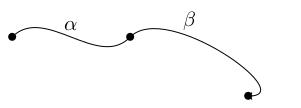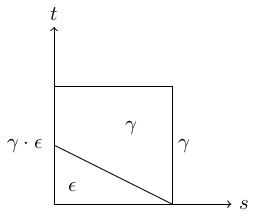# 1.03 Concatenation and the fundamental group

Below the video you will find accompanying notes and some pre-class questions.

# Notes

## Concatenation

(0.10) Let $$X$$ be a topological space and suppose $$\alpha,\beta\colon[0,1]\to X$$ are paths such that $$\alpha(1)=\beta(0)$$. The concatenation $$\beta\cdot\alpha$$ is the path $(\beta\cdot\alpha)(t)=\begin{cases}\alpha(2t)&\mbox{ if }t\in[0,1/2]\\\beta(2t-1)&\mbox{ if }t\in[1/2,1].\end{cases}$## The fundamental group

(3.49) Given a topological space $$X$$ and a basepoint $$x\in X$$, write $$\Omega_xX$$ for the set of all loops in $$X$$ based at $$x$$. The relation $$\simeq$$ that two loops $$\alpha,\beta\in\Omega_xX$$ are based-homotopic is an equivalence relation. We write $$\pi_1(X,x)$$ for the set of equivalence classes $$\Omega_xX/\simeq$$ of loops up to based homotopy.

We can make $$\pi_1(X,x)$$ into a group under concatenation: if $$[\alpha]\in\pi_1(X,x)$$ denotes the homotopy class of the loop $$\alpha$$ then \\alpha.\]

The operation $$[\beta]\cdot[\alpha]=[\beta\cdot\alpha]$$ defines a group structure on $$\pi_1(X,x)$$. In particular:
• it is well-defined on homotopy classes,
• it is associative,
• it has an identity (the identity element is the constant loop $$\epsilon(t)=x$$),
• each homotopy class of loops $$[\gamma]$$ has an inverse $$[\bar{\gamma}]$$, where $$\bar{\gamma}(t)=\gamma(1-t)$$ is the loop which runs around $$\gamma$$ in reverse.
(8.30) We will show that the constant loop $$\epsilon$$ is an identity for concatenation on $$\pi_1(X,x)$$; the other parts are left as exercises.

The concatenation $$\gamma\cdot\epsilon$$ stays at $$x$$ for time $$1/2$$ and then moves around $$\gamma$$ at double-speed. This is not /equal to/ the loop $$\gamma$$, but it only differs in the way it is parametrised. By playing with the parametrisation, we can construct a homotopy $$\gamma_s$$ from this concatenation to the original loop $$\gamma$$. $\gamma_s(t)=\begin{cases} \epsilon(t)&\mbox{ if }t\leq 1/2(1-s)\\ \gamma((2-s)t+s-1)&\mbox{ if }t\geq 1/2(1-s) \end{cases}$ (10.46) To understand this formula, observe that at the stage $$s$$ in the homotopy, the loop $$\gamma_s$$ stays at $$x$$ for time $$1/2(1-s)$$ ($$1/2$$ when $$s=0$$ and $$0$$ when $$s=1$$) then moves around $$\gamma$$ at $$(2-s)$$ times the speed of the original loop (twice as fast when $$s=0$$, the same speed as the original when $$s=1$$). Here is a picture of the domain of the homotopy:(14.46) This homotopy is continuous by one of the exercises we will do in class when we learn about topological spaces; it is a homotopy rel endpoints since $$\gamma_s(0)=\gamma_s(1)=x$$ for all $$s$$; and it satisfies $$\gamma_0=\gamma\cdot\epsilon$$ and $$\gamma_1=\gamma$$. A similar homotopy works for $$\epsilon\cdot\gamma$$.

# Pre-class questions

1. Suppose that $$\alpha_t$$ is a homotopy between $$\alpha_0$$ and $$\alpha_1$$ and $$\beta_t$$ is a homotopy between $$\beta_0$$ and $$\beta_1$$. Can you write down a homotopy between $$\beta_0\cdot\alpha_0$$ and $$\beta_1\cdot\alpha_1$$? This verifies one of the claims from the lemma in the video/notes: which claim?